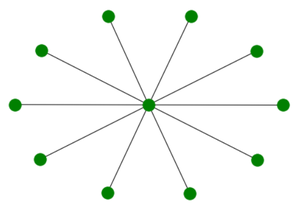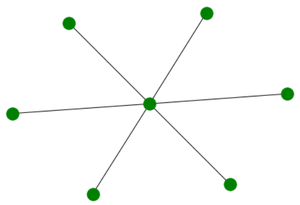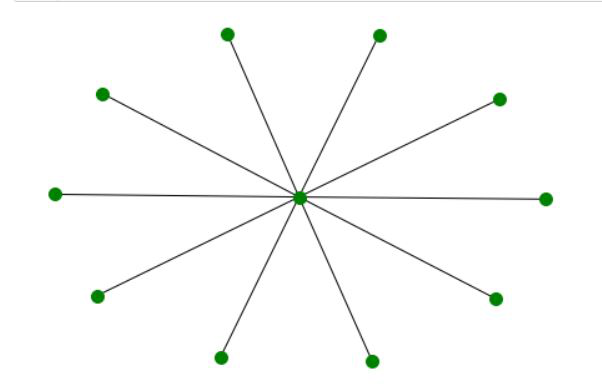Open in App
Not now

# Star Graph using Networkx Python

• Last Updated : 17 May, 2021

In this article, we are going to see Star Graph using Networkx Python. A Star graph is a special type of graph in which n-1 vertices have degree 1 and a single vertex have degree n – 1. This looks like that n – 1 vertex is connected to a single central vertex. A star graph with total n – vertex is termed as Sn.

Properties of Star Graph:

• It has n+1 vertices.
• It has n edges.
• It does not have any cycle.
• The diameter of a star graph Sn is a minimum of (2, n).
• A Star graph is a tree.
• It has no unconnected component.
• The chromatic number of the star graph is a minimum of (2, n + 1).

Example of S10 :S10

Example of S6 :S6

Approach:

• We will import the required networkx module
• After that, we will initialize a number of nodes to 6.
• We will create graph object G using star_graph() function.
• We will realize the graph using nx.draw() function.
• We will make the color of nodes green and increasing size by passing extra arguments to nx.draw().

Example 1:

## Python3

 `# import required module``import` `networkx as nx``  ` `# create object``G ``=` `nx.star_graph(``6``)``  ` `# illustrate graph``nx.draw(G, node_color ``=` `'green'``,``        ``node_size ``=` `100``)`

Output:Explanation:

As we passed 6 as an argument to the star_graph() function hence we got a star graph with 6 edges as output. We changed the color and size of nodes by passing extra arguments node_size and node_color to the nx.draw() function.

Example 2:

## Python3

 `# import required module``import` `networkx as nx``  ` `# create object``G ``=` `nx.star_graph(``10``)``  ` `# illustrate graph``nx.draw(G, node_color ``=` `'green'``,``        ``node_size ``=` `100``)`

Output:Explanation:

As we passed 10 as an argument to the star_graph() function hence we got a star graph with 10 edges as output. We changed the color and size of nodes by passing extra arguments node_size and node_color to the nx.draw() function.

My Personal Notes arrow_drop_up